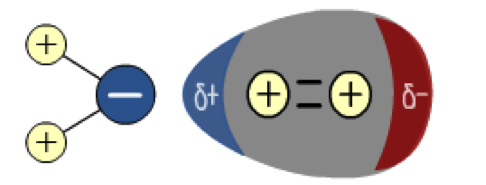Determining Water Purity via Biological Oxygen Demand

Prior to studying this section, you may wish to revise your solution calculations and your molar calculations.

Aquatic life is dependent upon gases dissolved in the water that they live in. Gases, such as carbon dioxide (CO2) will be required by aquatic plants for photosynthesis, whereas most aquatic plants and animals require oxygen for anaerobic respiration. Microorganisms will also require oxygen as they go about decomposing organic matter. One important indicator of water quality is the dissolved oxygen content of the water. At a pressure of 1atm and a temperature of 20oC, the maximum solubility of oxygen is about 9ppm, which equates to 0.009 g dm-3. Oxygen, being a non-polar molecule, has a low solubility in water, which is a polar solvent. The polar water molecule induces a dipole moment on the oxygen molecule and the two molecules are now weakly attracted.

The diagram below shows the polar water molecule on the left inducing a dipole moment on the non-polar oygen molecule leading to a weak force of attraction;To ensure a balanced and diverse aquatic community, the oxygen content should not fall below 6ppm, although some species of fish can survive in environments with oxygen contents as low as 3ppm. Bacteria are able to survive in water with even lower levels of oxygen.

When organic matter decomposes aerobically in water, the bacteria responsible for this process use up some of the dissolved oxygen present in the water. The amount of oxygen required by the bacteria to decompose this organic matter is defined as biological oxygen demand (BOD). This is often measured in a fixed volume of water over a fixed period of time such as 5 days.

If water has a high BOD without the means of replenishing the used oxygen, then very soon it will not be able to support aquatic life. There is a high risk of this happening in bodies of water that are still and do not have much mechanical mixing, e.g. lakes and ponds, whereas fast flowing rivers are able to replenish this oxygen via the mechanical action of its flow.

So what factors could cause an increase in BOD? If excessive biodegradable materials find their way into water, this will lead to an increase in decomposing bacteria and hence an increase in BOD. Possible sources of this material include sewerage and industrial wastes from food processing or paper mills. BOD of water can also increase due to the addition of nutrients such as nitrates and phosphates that can be found in fertilizers or laundry detergents. This causes an increase in algae growth, which eventually dies, and decays. As plant growth becomes excessive, the volume of dead and decaying organic material increases rapidly. This decay requires O2, leading to the depletion of O2 in the water.

We can use BOD values as an indicator of water quality.

 BOD/ppm Quality of water < 1 Almost pure water 5 Doubtful purity 10 Unacceptable quality 100 - 400 Waste from untreated sewerage 100 - 10000 Waste from meat processing
The BOD of a sample of water can be tested using a redox titration called the Winkler method. The principle of the Winkler method is as follows; oxygen in a water sample is made to oxidise iodide ions into iodine. The amount of iodine produced is determined by titrating with a standard thiosulphate solution. The amount of oxygen present in the original sample of water can be determined from the titer.

The reactions are summarized as follows:

1) 2 Mn2+(aq) + 4 OH-(aq) + O2(aq) → 2 MnO2(s) + 2 H2O(l)

2) MnO2(s) + 4 H+(aq) + 2 I-(aq) → Mn2+(aq) + I2(aq) + 2 H2O(l)

3) I2(aq) + 2 S2O32-(aq) → S4O62-(aq) + 2 I-(aq)

Using the steps outlined above, please calculate the following.

A 500 cm3 sample of water was saturated with oxygen and left for 5 days. The final oxygen content was measured using the sequence of reactions highlighted above. It was found that 5.00cm3 of a 0.0500 mol dm-3 solution of Na2S2O3(aq) was required to react with the iodine produced.

a) Calculate how many moles of Na2S2O3(aq) reacted with the iodine in reaction (3)

b) Deduce how many moles of iodine had been produced in reaction (2).

c) Deduce how many moles of MnO2(s) had been produced in reaction (1).

d) Deduce how many moles of O2(g) were present in the water.

e) Calculate the solubility of oxygen in the water in g dm-3.

f) Assume the maximum solubility of the water is 0.009 g dm-3 and deduce the BOD of the water sample.

Solution

a) Amount of Na2S2O3(aq) = 5.00 x 0.0500/1000 = 2.5 x 10-4 moles.

b) Amount of I2(aq) = ½ (2.50 x 10-4 moles) = 1.25 x 10-4 moles.

c) Amount of MnO2(s) = 1.25 x 10-4 moles.

d) Amount of O2(g) = ½ (1.25 x 10-4) moles = 6.25 x 10-5 moles.

e) Amount of O2(g) in 1dm3 = 1.25 x 10-4 moles Mass in 1dm3 = 0.004 g dm-3

f) Oxygen used by bacteria (BOD) = 0.009 g dm-3 – 0.004 g dm-3 = 0.005 g dm-3